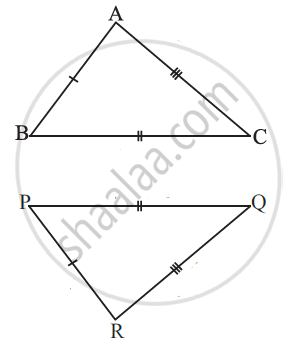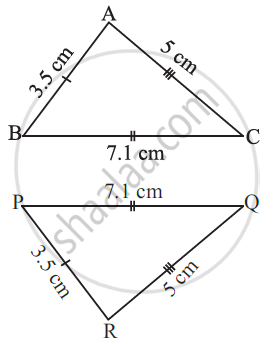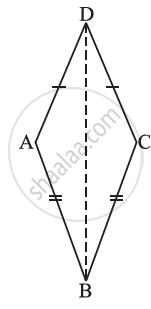# Criteria for Congruence of Triangles - SSS Congruence Criterion

## Definition

SSS Congruence criterion: If under a given correspondence, the three sides of one triangle are equal to the three corresponding sides of another triangle, then the triangles are congruent.

## Notes

### SSS Congruence criterion:

If under a given correspondence, the three sides of one triangle are equal to the three corresponding sides of another triangle, then the triangles are congruent.Side AB = Side PR

Side BC = Side PQ

Side AC = Side RQ

A ↔ R, B ↔ P, and C ↔ Q.

Thus, △ ABC ≅ △ RPQ............(SSS Congruence criterion)

## Example

In triangles ABC and PQR, AB = 3.5 cm, BC = 7.1 cm, AC = 5 cm, PQ = 7.1 cm, QR = 5 cm and PR = 3.5 cm. Examine whether the two triangles are congruent or not. If yes, write the congruence relation in symbolic form.Here,
AB = PR (= 3.5 cm),
BC = PQ (= 7.1 cm) and
AC = QR (= 5 cm)

This shows that the three sides of one triangle are equal to the three sides of the other triangle. So, by SSS congruence rule, the two triangles are congruent. From the above three equality relations, it can be easily seen that A ↔ R, B ↔ P, and C ↔ Q.

So, we have ∆ ABC ≅ ∆ RPQ.

## Example

In Fig, AD = CD and AB = CB.(i) State the three pairs of equal parts in ∆ABD and ∆CBD.
(ii) Is ∆ABD ≅ ∆CBD? Why or why not?
(iii) Does BD bisect ∠ABC? Give reasons.

(i) In ∆ABD and ∆CBD, the three pairs of equal parts are as given below:
AB =CB.....................(Given)
BD =BD....................(Common in both)

(ii) From (i) above, ∆ABD ≅ ∆CBD.............(By SSS congruence rule)

(iii) ∠ABD = ∠CBD..................(Corresponding parts of congruent triangles)
So, BD bisects ∠ABC.

If you would like to contribute notes or other learning material, please submit them using the button below.

### Shaalaa.com

SSS Congruence Criterion [00:10:24]
S
0%